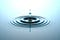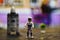# Riding the Wave, Numerically.

A brief introduction to finite-difference method and its application to solve PDEs numerically.Image source

Universe is a complex entity where various natural phenomena are governed by equations which depend on multiple variables and have non-linear behavior. These equations can take form of ordinary or partial differential equations. In the previous article, we saw the numerical integration method to solve Ordinary Differential Equations(ODEs) numerically. In this article, we will try to understand what Partial Differential Equations (PDEs) actually are and then use finite-difference method to solve a physical phenomenon. In the process, we will also learn some extensive python functions and libraries.

# Flying A Rocket, Numerically.

## A brief introduction to simulations and converting discretized Ordinary Differential Equations into a python code to model a physical phenomenon.Photo by Phil Hearing on Unsplash

The Universe is continuous or discrete is up for a debate (Greek philosopher Zeno’s glance over two giants: General theory of relativity & Quantum theory). Nonetheless, a physical phenomenon such as flow of an inviscid fluid even though seems continuous from a distance, we still need to discretize them in order to quantitatively ‘measure’ any of its property. And when we, humans ( we will keep an Earthling’s perspective in this article ), measure something we always lose some accuracy in the process. Isn’t it surprising? We shall get back to this a little later.

Studying a physical phenomena with…## Sidhant Jain

Just a Computational Physicist. Failed PhD.